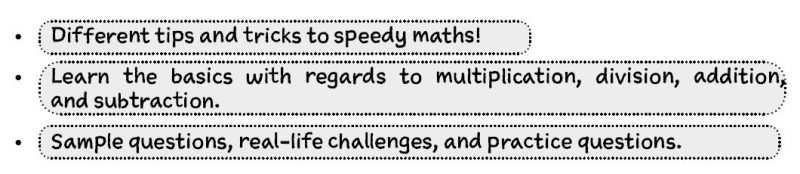## QUICK-FIRE MATHS

Rapid Study Skills for Students: Quick-Fire Maths contains top tricks and secrets to increase your mathematical speed.

Open up this guide to discover how you can increase your mathematical skills by learning some neat little tricks which will ultimately save time.

This easy-to-absorb resource has been designed to help those master the basics of Maths using these rapid maths tricks. Packed full of tips, tricks and sample questions, this compact guide is ideal for anyone to improve their mathematical learning and speed.How Will This Guide Benefit Me?
Not only will you learn an array of techniques that will benefit your mathematical learning, but you will also be able to learn NEW ways of working out basic addition, subtraction, multiplication, and division calculations.

This guide has been carefully designed to guide you through some of the most useful mathematical techniques. Within this guide, you will be taught the following:Sample Tips and Tricks
Let’s have a look at some of the tips and tricks included in this guide:

You can subtract any number without even having to subtract any numbers at all. Sounds weird, doesn’t it? But it can be done!MULTIPLICATION BY 5
The key to this technique is to divide the number by 2 and then insert any necessary zeroes.DIVISION BY 25, 2.5, 250 and so on…
The trick to dividing any number by 25 is the following: Multiply the number by 4 and then affix any zeroes or decimal points where necessary.SQUARING A TWO-DIGIT NUMBER BEGINNING WITH 5
When it comes to squaring numbers, this is also another handy trick to learn.

This trick will work for ANY number beginning with 5. The technique is as follows: add 25 to the units digits. Using the units number, affix the square to it.

The key thing to remember is the following:
5 x 5 = 25ESTIMATION – DIVISION BY 14, 1.4, 140 and so on…
When it comes to estimating an answer with regards to dividing by 14, you can multiply the number by 7, and then affix any zeroes or decimal points if necessary.RAPID STUDY SKILLS FOR STUDENTS – QUICK-FIRE MATHS

Written and created by the UK’s leading recruitment experts; this handy pocketbook contains:

• Rapid Maths tricks and tips;
• Time-saving tricks to perform the most common maths calculations – without ever needing a calculator;
• Packed full of actionable tips and exercises;
• Increase your mathematical speed with ease!

Free Bonus ItemPlus 30-days FREE ACCESS to the Educational online testing suite. Thereafter, just £5.95 per month. No minimum term. You may cancel anytime. Cancel before the 30-days are up and you will not be charged. Online suite access on PC, MAC and mobile devices.

```
```

## Order Now

Improve your maths skills with this handy guide. Trusted by 10,000s of customers worldwide.

SPECIAL OFFER
INSTANT ACCESS
£4.99Includes:
✓ Rapid Maths tricks and tips;
✓ Time-saving tricks to perform the most common maths calculations;
✓ Learn in a concise manner with this easy-to- absorb pocketbook, full of actionable tips and exercises;
✓ Increase your mathematical speed with ease!
✓ An ideal book for anyone who wants to improve their maths skills.

30-Day Money-back Guarantee